• 图像处理中高通滤波与低通滤波 High pass filter A high pass filter (HPF) is a filter that examines a region of an image and boosts the intensity of certain pixels based on the difference...
                          图像处理中的高通滤波与低通滤波

High pass filter

A high pass filter (HPF) is a filter that examines a region of an image and boosts

the intensity of certain pixels based on the difference in the intensity with the

surrounding pixels.

Take, for example, the following kernel:

[[0, -0.25, 0],

[-0.25, 1, -0.25],

[0, -0.25, 0]]

After calculating the sum of differences of the intensities of the central pixel

compared to all the immediate neighbors, the intensity of the central pixel will be

boosted (or not) if a high level of changes are found. In other words, if a pixel stands

out from the surrounding pixels, it will get boosted.

This is particularly effective in edge detection, where a common form of HPF called

high boost filter is used.

Here, the pixel of interest has a weight of 9 and its immediate neighbors each

have a weight of -1. For the pixel of interest, the output color will be nine times

its input color minus the input colors of all eight adjacent pixels. If the pixel

of interest is already a bit different from its neighbors, this difference becomes

intensified. The effect is that the image looks sharper as the contrast between the

neighbors is increased.

Note that both filters sum up to 0, the reason for this is

explained in detail in the Edge detection section.

If we modify a sharpening kernel slightly so that its weights sum up to 0 instead, we have an edge detection kernel that turns edges white and non-edges black.

array([[-1, -1, -1],

[-1, 9, -1],

[-1, -1, -1]])

这个模板其权重之和为1.在图像非高频部分作用于该模板之后，中心像素所得的灰度值总是非零灰度值，和周边像素一样；而高频部分作用于改模板之后，中心像素的灰度值得到了增强，但也是非零灰度值。对于查找边缘或锐化而言，是无法区分图像的边缘或图像的高频部分。

所以，修改该模板使得其权重之和为0.这样边缘部分经过该模板之后，中心像素变成白色或者说有灰度值；非边缘部分经过该模板卷积之后，中心像素的灰度值变为0，这样就可以很好的区别边缘和非边缘部分了。2019.1.11

Low pass filter

If an HPF boosts the intensity of a pixel, given its difference with its neighbors, a

low pass filter (LPF) will smoothen the pixel if the difference with the surrounding

pixels is lower than a certain threshold. This is used in denoising and blurring. For

example, one of the most popular blurring/smoothening filters, the Gaussian blur,

is a low pass filter that attenuates the intensity of high frequency signals.

Take, for example, the following kernel:

以上是均值滤波模板，各个权重权重值都相等。对于高斯滤波而言，模板中靠近中心像素的权重值比远离中心像素的权重值要大，但是其所有权重值相加之后也应该也是为1.因为高斯滤波作为低通滤波器而言，仍然需要将中心像素灰度值向邻域内的像素的灰度值靠拢。

图像中的边缘、细节部分属于图像的高频信息；图像的非边缘、细节部分属于图像的低频信息。高通滤波器的作用顾名思义，就是可以让高频信号通过的滤波器，低通滤波器就是让低频信号通过的滤波器。

在上述高通滤波器模板中，如果中心像素的灰度值大于邻域内其他像素，那么中心像素通过高通滤波器之后其灰度值将被增加；反之，低通滤波器中，如果中心像素的灰度值大于邻域其他像素的灰度值，那么中心像素的灰度值将会向周边邻域内像素灰度值大小靠拢。


展开全文图像处理 低通滤波
• 高通滤波处理案例： 原始图片 高通滤波 增强型高通滤波 一般在高通滤波之后，在灰度级窄范围以灰度为特征的图像可以用直方图均衡化来进一步的增强。
高通滤波的处理案例：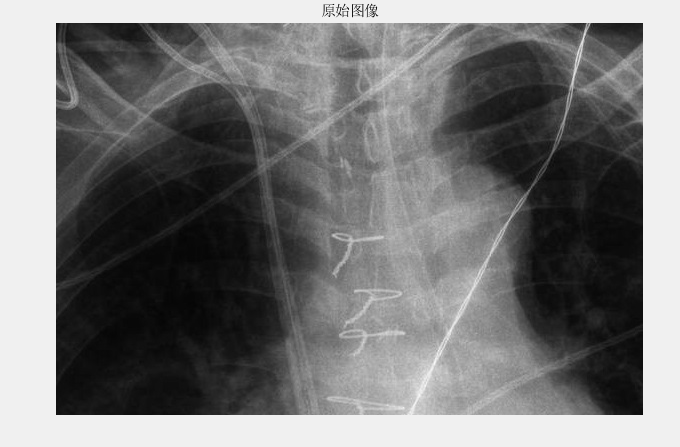原始图片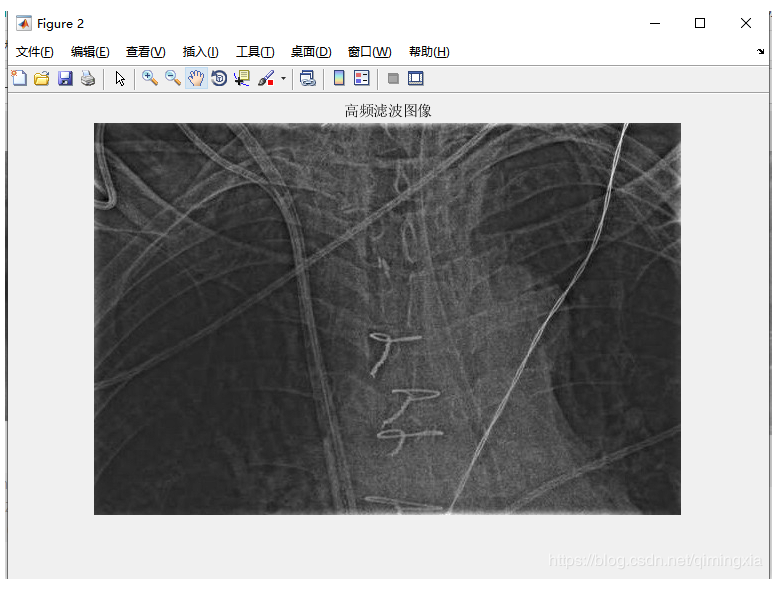高通滤波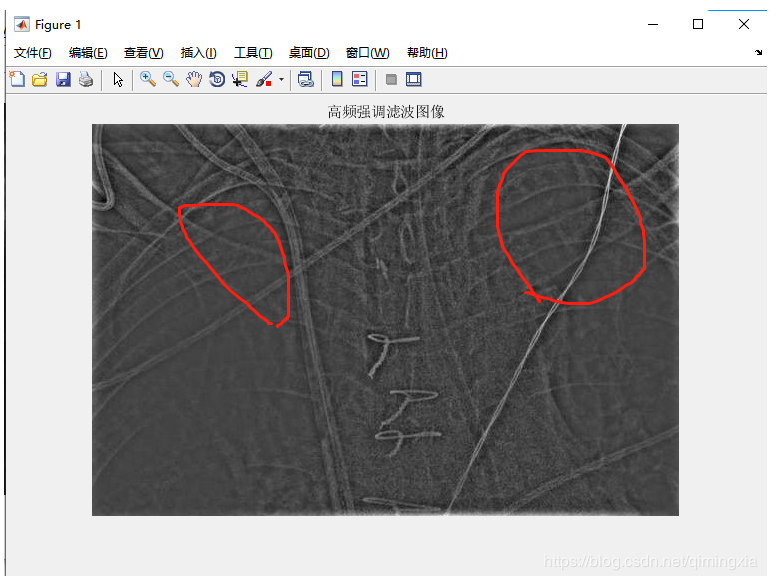增强型高通滤波
一般在高通滤波之后，在灰度级窄范围以灰度为特征的图像可以用直方图均衡化来进一步的增强。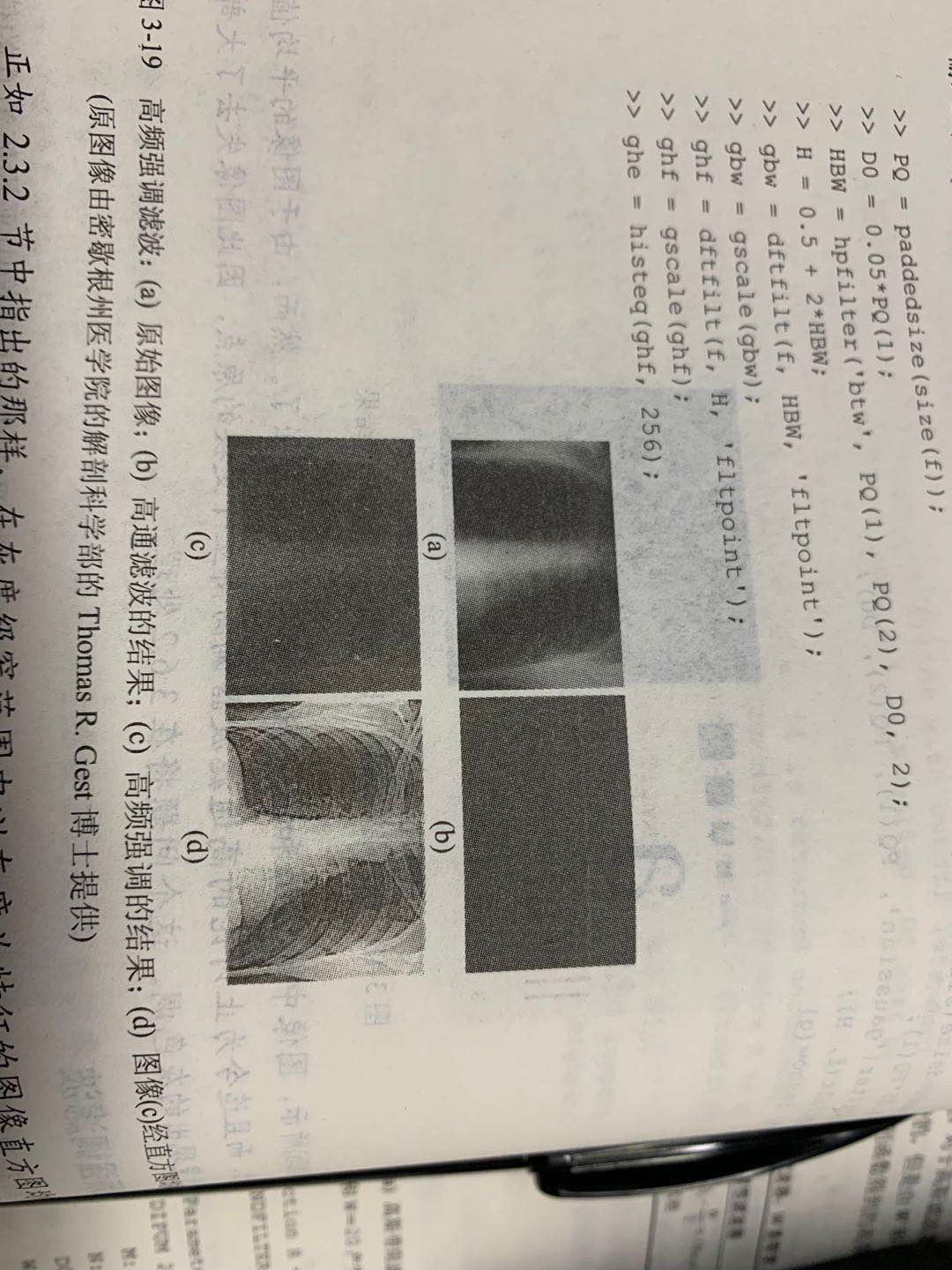展开全文matlab
• 前面一篇文章我讲解了傅里叶变换，它将时间域上的信号转变为频率域上的信号，用来进行图像除噪、图像增强等处理。本文将继续补充基于傅里叶变换的高通滤波和低通滤波。基础性文章，希望对你有所帮助。同时，该部分...
该系列文章是讲解Python OpenCV图像处理知识，前期主要讲解图像入门、OpenCV基础用法，中期讲解图像处理的各种算法，包括图像锐化算子、图像增强技术、图像分割等，后期结合深度学习研究图像识别、图像分类应用。希望文章对您有所帮助，如果有不足之处，还请海涵~
该系列在github所有源代码：https://github.com/eastmountyxz/ImageProcessing-Python
PS：请求帮忙点个Star，哈哈，第一次使用Github，以后会分享更多代码，一起加油。
同时推荐作者的C++图像系列知识：
[数字图像处理] 一.MFC详解显示BMP格式图片
[数字图像处理] 二.MFC单文档分割窗口显示图片
[数字图像处理] 三.MFC实现图像灰度、采样和量化功能详解
[数字图像处理] 四.MFC对话框绘制灰度直方图
[数字图像处理] 五.MFC图像点运算之灰度线性变化、灰度非线性变化、阈值化和均衡化处理详解
[数字图像处理] 六.MFC空间几何变换之图像平移、镜像、旋转、缩放详解
[数字图像处理] 七.MFC图像增强之图像普通平滑、高斯平滑、Laplacian、Sobel、Prewitt锐化详解
前文参考：
[Python图像处理] 一.图像处理基础知识及OpenCV入门函数
[Python图像处理] 二.OpenCV+Numpy库读取与修改像素
[Python图像处理] 三.获取图像属性、兴趣ROI区域及通道处理
[Python图像处理] 四.图像平滑之均值滤波、方框滤波、高斯滤波及中值滤波
[Python图像处理] 五.图像融合、加法运算及图像类型转换
[Python图像处理] 六.图像缩放、图像旋转、图像翻转与图像平移
[Python图像处理] 七.图像阈值化处理及算法对比
[Python图像处理] 八.图像腐蚀与图像膨胀
[Python图像处理] 九.形态学之图像开运算、闭运算、梯度运算
[Python图像处理] 十.形态学之图像顶帽运算和黑帽运算
[Python图像处理] 十一.灰度直方图概念及OpenCV绘制直方图
[Python图像处理] 十二.图像几何变换之图像仿射变换、图像透视变换和图像校正
[Python图像处理] 十三.基于灰度三维图的图像顶帽运算和黑帽运算
[Python图像处理] 十四.基于OpenCV和像素处理的图像灰度化处理
[Python图像处理] 十五.图像的灰度线性变换
[Python图像处理] 十六.图像的灰度非线性变换之对数变换、伽马变换
[Python图像处理] 十七.图像锐化与边缘检测之Roberts算子、Prewitt算子、Sobel算子和Laplacian算子
[Python图像处理] 十八.图像锐化与边缘检测之Scharr算子、Canny算子和LOG算子
[Python图像处理] 十九.图像分割之基于K-Means聚类的区域分割
[Python图像处理] 二十.图像量化处理和采样处理及局部马赛克特效
[Python图像处理] 二十一.图像金字塔之图像向下取样和向上取样
[Python图像处理] 二十二.Python图像傅里叶变换原理及实现
前面一篇文章我讲解了傅里叶变换，它将时间域上的信号转变为频率域上的信号，用来进行图像除噪、图像增强等处理。本文将继续补充基于傅里叶变换的高通滤波和低通滤波。基础性文章，希望对你有所帮助。同时，该部分知识均为杨秀璋查阅资料撰写，转载请署名CSDN+杨秀璋及原地址出处，谢谢！！
1.高通滤波
2.低通滤波
3.本章小结

PS：文章参考自己以前系列图像处理文章及OpenCV库函数，同时参考如下文献：
《数字图像处理》（第3版），冈萨雷斯著，阮秋琦译，电子工业出版社，2013年.
《数字图像处理学》（第3版），阮秋琦，电子工业出版社，2008年，北京.
《OpenCV3编程入门》，毛星云，冷雪飞，电子工业出版社，2015，北京.
百度百科-傅里叶变换
网易云课堂-高登教育 Python+OpenCV图像处理

一.高通滤波
傅里叶变换的目的并不是为了观察图像的频率分布（至少不是最终目的），更多情况下是为了对频率进行过滤，通过修改频率以达到图像增强、图像去噪、边缘检测、特征提取、压缩加密等目的。
过滤的方法一般有三种：低通（Low-pass）、高通（High-pass）、带通（Band-pass）。所谓低通就是保留图像中的低频成分，过滤高频成分，可以把过滤器想象成一张渔网，想要低通过滤器，就是将高频区域的信号全部拉黑，而低频区域全部保留。例如，在一幅大草原的图像中，低频对应着广袤且颜色趋于一致的草原，表示图像变换缓慢的灰度分量；高频对应着草原图像中的老虎等边缘信息，表示图像变换较快的灰度分量，由于灰度尖锐过度造成
高通滤波器是指通过高频的滤波器，衰减低频而通过高频，常用于增强尖锐的细节，但会导致图像的对比度会降低。该滤波器将检测图像的某个区域，根据像素与周围像素的差值来提升像素的亮度。图展示了“Lena”图对应的频谱图像，其中心区域为低频部分。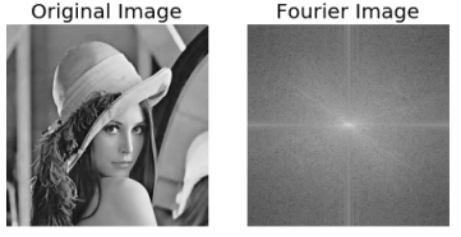接着通过高通滤波器覆盖掉中心低频部分，将255两点变换为0，同时保留高频部分，其处理过程如下图所示。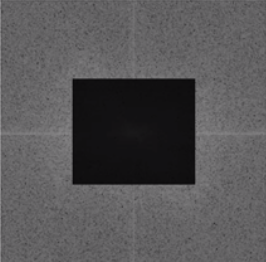rows, cols = img.shape
crow,ccol = int(rows/2), int(cols/2)
fshift[crow-30:crow+30, ccol-30:ccol+30] = 0

通过高通滤波器将提取图像的边缘轮廓，生成如下图所示图像。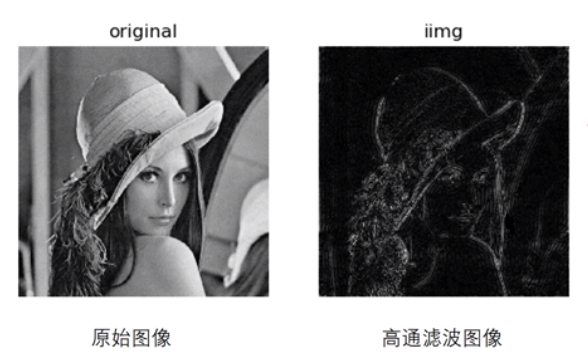# -*- coding: utf-8 -*-
import cv2 as cv
import numpy as np
from matplotlib import pyplot as plt

#读取图像

#傅里叶变换
f = np.fft.fft2(img)
fshift = np.fft.fftshift(f)

#设置高通滤波器
rows, cols = img.shape
crow,ccol = int(rows/2), int(cols/2)
fshift[crow-30:crow+30, ccol-30:ccol+30] = 0

#傅里叶逆变换
ishift = np.fft.ifftshift(fshift)
iimg = np.fft.ifft2(ishift)
iimg = np.abs(iimg)

#显示原始图像和高通滤波处理图像
plt.subplot(121), plt.imshow(img, 'gray'), plt.title('Original Image')
plt.axis('off')
plt.subplot(122), plt.imshow(iimg, 'gray'), plt.title('Result Image')
plt.axis('off')
plt.show()

输出结果如下图所示，第一幅图为原始“Lena”图，第二幅图为高通滤波器提取的边缘轮廓图像。它通过傅里叶变换转换为频谱图像，再将中心的低频部分设置为0，再通过傅里叶逆变换转换为最终输出图像“Result Image”。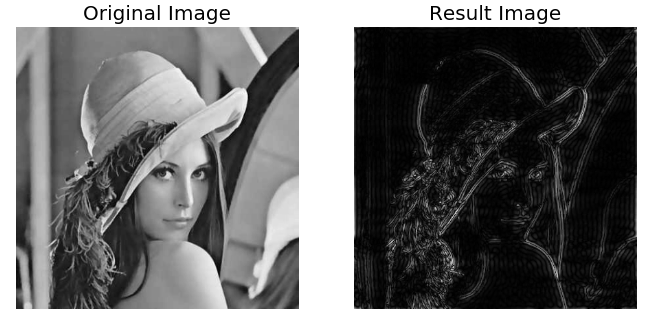二.低通滤波
低通滤波器是指通过低频的滤波器，衰减高频而通过低频，常用于模糊图像。低通滤波器与高通滤波器相反，当一个像素与周围像素的插值小于一个特定值时，平滑该像素的亮度，常用于去燥和模糊化处理。如PS软件中的高斯模糊，就是常见的模糊滤波器之一，属于削弱高频信号的低通滤波器。
下图展示了“Lena”图对应的频谱图像，其中心区域为低频部分。如果构造低通滤波器，则将频谱图像中心低频部分保留，其他部分替换为黑色0，其处理过程如图所示，最终得到的效果图为模糊图像。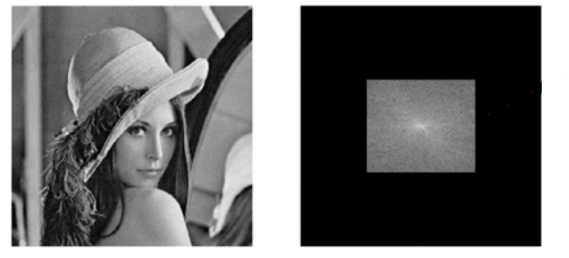那么，如何构造该滤波图像呢？如下图所示，滤波图像是通过低通滤波器和频谱图像形成。其中低通滤波器中心区域为白色255，其他区域为黑色0。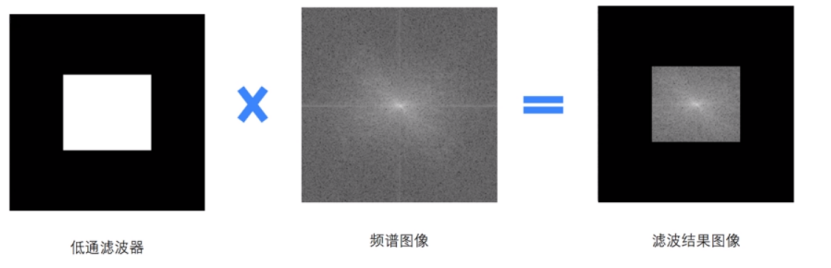低通滤波器主要通过矩阵设置构造，其核心代码如下：
rows, cols = img.shape
crow,ccol = int(rows/2), int(cols/2)
mask = np.zeros((rows, cols, 2), np.uint8)

通过低通滤波器将模糊图像的完整代码如下所示：
# -*- coding: utf-8 -*-
import cv2
import numpy as np
from matplotlib import pyplot as plt

#读取图像

#傅里叶变换
dft = cv2.dft(np.float32(img), flags = cv2.DFT_COMPLEX_OUTPUT)
fshift = np.fft.fftshift(dft)

#设置低通滤波器
rows, cols = img.shape
crow,ccol = int(rows/2), int(cols/2) #中心位置
mask = np.zeros((rows, cols, 2), np.uint8)

#掩膜图像和频谱图像乘积

#傅里叶逆变换
ishift = np.fft.ifftshift(f)
iimg = cv2.idft(ishift)
res = cv2.magnitude(iimg[:,:,0], iimg[:,:,1])

#显示原始图像和低通滤波处理图像
plt.subplot(121), plt.imshow(img, 'gray'), plt.title('Original Image')
plt.axis('off')
plt.subplot(122), plt.imshow(res, 'gray'), plt.title('Result Image')
plt.axis('off')
plt.show()

输出结果如图所示，第一幅图为原始“Lena”图，第二幅图为低通滤波器模糊处理后的图像。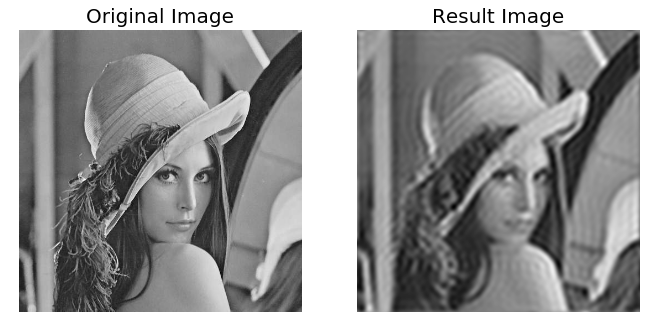三.总结
讲到这里，傅里叶变换的图像增强、图像去噪、边缘检测、特征提取已介绍，前面的文章详细讲解了图像平滑和图像锐化各种算子，希望读者能进行相关对比。下一篇文章，作者将分享几个Python图像处理的特效处理，包括素描、黄昏、灯光、浮雕等效果，希望读者喜欢。
时也，命也。
英语低分数线一分，些许遗憾，但不气馁，更加努力。雄关漫道真如铁，而今迈过从头越，从头越。苍山如海，残阳如血。感谢一路陪伴的人和自己。
无论成败，那段拼搏的日子都很美。结果只会让我更加努力，学好英语。下半年沉下心来好好做科研写文章，西藏之行，课程分享。同时，明天的博士考试加油，虽然裸泳，但也加油！还有春季招考开始准备。
最后补充马刺小石匠精神，当一切都看起来无济于事的时候，我去看一个石匠敲石头．他一连敲了100次，石头仍然纹丝不动。但他敲第101次的时候，石头裂为两半。可我知道，让石头裂开的不是那最后一击，而是前面的一百次敲击的结果。人生路漫漫，不可能一路一帆风顺，暂时的不顺只是磨练自己的必经之路，夜最深的时候也是距黎明最近的时刻，经历过漫漫长夜的打磨，你自身会更加强大。
最后希望这篇基础性文章对您有所帮助，如果有错误或不足之处，请海涵！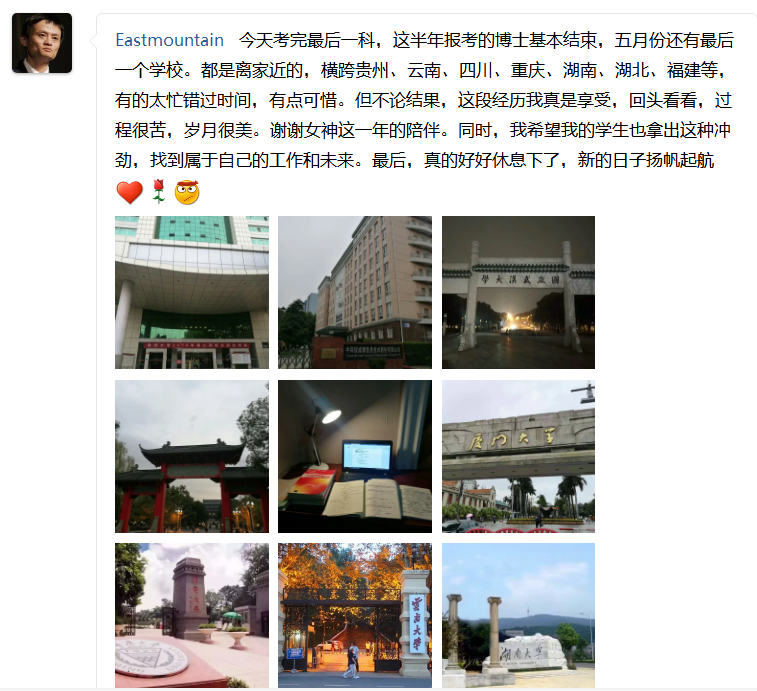（By：Eastmount 2019-04-28 深夜96点写于花溪 https://blog.csdn.net/Eastmount )


展开全文Python图像处理 傅里叶变换 高通滤波 低通滤波
• 高通滤波：边缘提取与增强低通滤波：边缘平滑边缘区域的灰度变换加大，也就是频率较高。所以，对于高通滤波，边缘部分将被保留，非边缘部分将被过滤；对于低通滤波，边缘区域将被平滑过渡。
高通滤波：边缘提取与增强

低通滤波：边缘平滑

边缘区域的灰度变换加大，也就是频率较高。所以，对于高通滤波，边缘部分将被保留，非边缘部分将被过滤；对于低通滤波，边缘区域将被平滑过渡。
展开全文图像处理
• 图像的频域滤波-高通滤波介绍 这里的高通滤波主要是巴特沃兹高通和理想高通，其频率特性正好和对应的低通相反，这里就不再赘述。 效果图 可见，理想高通仍有振铃效应的产生，而巴特沃兹就好很多。 加强滤波是为了...
图像的频域滤波-高通滤波

介绍

这里的高通滤波主要是巴特沃兹高通和理想高通，其频率特性正好和对应的低通相反，这里就不再赘述。

效果图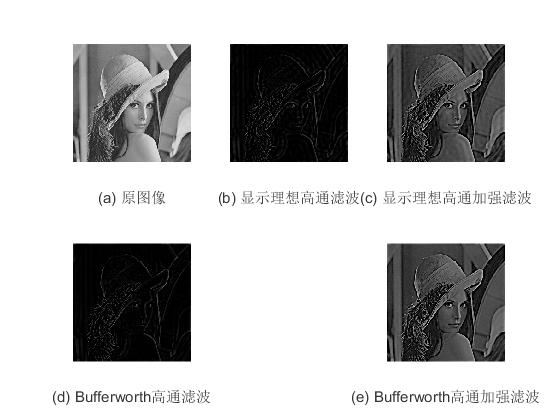可见，理想高通仍有振铃效应的产生，而巴特沃兹就好很多。
加强滤波是为了弥补一下低频信息，让图像看上去更有意思一点哈。

示例代码

clc;
clear all;
close all;
if size(J, 3)==3
J = rgb2gray(J);
end
subplot(2,3,1);imshow(uint8(J));
xlabel('(a) 原图像');

J=double(J);
f=fft2(J);      %采用傅里叶变换
g=fftshift(f);   %数据矩阵平衡
[M,N]=size(f);
n1=floor(M/2);
n2=floor(N/2);
d0=20;
for i=1:M    %进行理想高通滤波和理想高通加强滤波
for j=1:N
d=sqrt((i-n1)^2+(j-n2)^2);
if d>=d0
h1=1;
h2=1+0.5;
else
h1=0;
h2=0.5;
end
g1(i,j)=h1*g(i,j);
g2(i,j)=h2*g(i,j);
end
end
g1=ifftshift(g1);
g1=uint8(real(ifft2(g1)));
subplot(2,3,2);imshow(g1);  %显示理想高通滤波结果
xlabel('(b) 显示理想高通滤波');
g2=ifftshift(g2);
g2=uint8(real(ifft2(g2)));
subplot(2,3,3);imshow(g2); %显示理想高通加强滤波结果
xlabel('(c) 显示理想高通加强滤波');
n=2;
d0=20;
for i=1:M        %进行Bufferworth高通滤波和Bufferworth高通加强滤波
for j=1:N
d=sqrt((i-n1)^2+(j-n2)^2);
if d==0
h1=0;
h2=.5;
else
h1=1/(1+(d0/d)^(2*n));
h2=1/(1+(d0/d)^(2*n))+0.5;
end
gg1(i,j)=h1*g(i,j);
gg2(i,j)=h2*g(i,j);
end
end
gg1=ifftshift(gg1);
gg1=uint8(real(ifft2(gg1)));
subplot(2,3,4);imshow(gg1);   %显示Bufferworth高通滤波
xlabel('(d) Bufferworth高通滤波');
gg2=ifftshift(gg2);
gg2=uint8(real(ifft2(gg2)));
subplot(2,3,6);imshow(gg2);   %显示Bufferworth高通加强滤波
xlabel('(e) Bufferworth高通加强滤波');

展开全文图像的高通滤波
• 如果想对图像进行滤波的话，基本的步骤如下： 使用(−1)x+y(-1)^{x+y}(−1)x+y乘以原来的图像，其中x、yx、yx、y是原图像的像素点的坐标。 对第一步得到的数据的进行离散傅里叶变换F(u,v)F(u,v)F(u,v) 使用某个...计算机视觉 opencv
• 结果如下 ...%而且至今为止，高通滤波器偏离了傅里叶变换的原点 图像失去了大部分原图像所呈现的背景色彩 %这个问题在高频强调滤波器讲明（下一个博客） f=imread('D：\亮度调节与空间滤波...图像处理
• import numpy as np import cv2 def convert_2d(r): r_ext = np.zeros((r.shape * 2, r.shape * 2)) for i in range(r.shape): for j in range(r.shape): r_ext[i][j] = r...数字图像处理 高通滤波
• 图像处理的matlab代码：Matlab实现图像高通滤波Matlab 图像高通滤波
• 图像中图像的边缘对应高频分量，因此高通滤波的效果是图像锐化。同样最简单的高通滤波器是理想高通滤波器。通过设置一个频率阈值，将高于该阈值的频率部分通过，而低于阈值的低频部分设置为0。 VTK理想高通滤波...
• 一、基于高通滤波融合算法原理 HPF融合算法原理其实就是将高空间分辨率全色影像的高频信息(细节、边缘)叠加到低分辨率多光谱影像上。其处理流程如下图所示，先将高分辨率全色影像与低分辨率多光谱影像进行直方图...图像融合
• 在笔记（七），我们通过衰减图像的傅里叶变换的高频成分来平滑对象，因为边缘和其他灰度的急剧变化与高频分量有关，所以图像的锐化可在频域通过高通滤波来实现。 一个高通滤波器是从给定的低通滤波器用下式得到： ...
• 理想高通滤波 H(u,v)={0,D(u,v)≤D01,D(u,v)>D0(14-1) H(u,v) = \begin{cases} 0 &, D(u,v) \leq D_0 \\ 1 &, D(u,v) \gt D_0 \end{cases} \tag{14-1} H(u,v)={01​,D(u,v)≤D0​,D(u,v)>D0...图像处理 图像降噪 机器视觉 计算机视觉
• 在此，使用高通滤波，提取图像边缘，进而锐化图像。highpass filter & sharpen image 与低通滤波器相反，高通滤波器允许图像高频部分通过，而高频部分证实图像的边缘信息（或者噪声）。得到如下的图像 sharpen ...高通滤波器 图像增强
• 巴特沃斯高通滤波 n阶巴特沃斯高通滤波的传递函数如下： H(u,v)=1−11+(D(u,v)/D0)2n(7-1) H(u,v) = 1 - \frac{1}{1 + (D(u,v) / D_0)^{2n}} \tag{7-1} H(u,v)=1−1+(D(u,v)/D0​)2n1​(7-1) 其中，D(u,v)=(u−P2)2+...图像处理 图像降噪 高通滤波 机器视觉 计算机视觉
• 《数字图像处理》冈萨雷斯 第三版，P179有一个指纹增强的例子； 先用opencv模拟实现： 处理这个指纹图像 ，其污染是很明显的。自动指纹识别的关键一步是增强指纹的脊线并减小污染。使用高通滤波实现； 脊线增强...
• 梯形高通滤波 梯形高通滤波器(TLPF)的传递函数如下：  H(u,v) = 1 - \begin{cases} 1 & D(u,v) \lt D_0 \\ \frac{D(u,v) - D_1}{D_0-D_1} & D_0 \lt D(u,v) \leq D_1 \\ 0 &D(u,v) > D_1 \end{...图像处理 图像降噪 高通滤波 机器视觉 计算机视觉
• 频域（与转移函数相乘、处理、傅里叶反变换）&gt;&gt;&gt;空域 2、常用频域增强方法： 巴特沃斯滤波器：阶为n，截断频率为D0的转移函数为： （1）低通滤波： 低通巴特沃斯滤波器在高低...
...